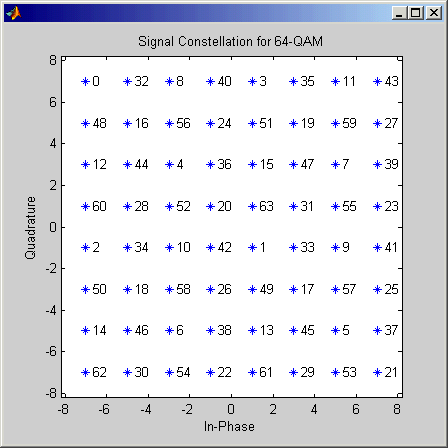# Rectangular QAM TCM Encoder

Convolutionally encode binary data and modulate using QAM method

## Library

TCM, in Digital Baseband sublibrary of Modulation

•## Description

The Rectangular QAM TCM Encoder block implements trellis-coded modulation (TCM) by convolutionally encoding the binary input signal and mapping the result to a QAM signal constellation.

The M-ary number parameter is the number of points in the signal constellation, which also equals the number of possible output symbols from the convolutional encoder. (That is, log2(M-ary number) is equal to n for a rate k/n convolutional code.)

### Input Signals and Output Signals

If the convolutional encoder described by the trellis structure represents a rate k/n code, then the Rectangular QAM TCM Encoder block's input must be a binary column vector with a length of L*k for some positive integer L.

The output from the Rectangular QAM TCM Encoder block is a complex column vector of length L.

### Specifying the Encoder

To define the convolutional encoder, use the Trellis structure parameter. This parameter is a MATLAB structure whose format is described in Trellis Description of a Convolutional Code. You can use this parameter field in two ways:

• If you want to specify the encoder using its constraint length, generator polynomials, and possibly feedback connection polynomials, then use a `poly2trellis` command within the Trellis structure field. For example, to use an encoder with a constraint length of 7, code generator polynomials of 171 and 133 (in octal numbers), and a feedback connection of 171 (in octal), set the Trellis structure parameter to

`poly2trellis(7,[171 133],171)`

• If you have a variable in the MATLAB workspace that contains the trellis structure, then enter its name as the Trellis structure parameter. This way is faster because it causes Simulink to spend less time updating the diagram at the beginning of each simulation, compared to the usage in the previous bulleted item.

The encoder registers begin in the all-zeros state. You can configure the encoder so that it resets its registers to the all-zeros state during the course of the simulation. To do this, set the `Operation mode` to Reset on nonzero input via port. The block then opens a second input port, labeled `Rst`. The signal at the `Rst` port is a scalar signal. When it is nonzero, the encoder resets before processing the data at the first input port.

### Signal Constellations

The trellis-coded modulation technique partitions the constellation into subsets called cosets, so as to maximize the minimum distance between pairs of points in each coset. This block internally forms a valid partition based on the value you choose for the M-ary number parameter.

The figures below show the labeled set-partitioned signal constellations that the block uses when M-ary number is `16`, `32`, and `64`. For constellations of other sizes, see Biglieri, E., D. Divsalar, P. J. McLane and M. K. Simon, Introduction to Trellis-Coded Modulation with Applications, New York, Macmillan, 1991.### Coding Gains

Coding gains of 3 to 6 decibels, relative to the uncoded case can be achieved in the presence of AWGN with multiphase trellis codes. For more information, see Biglieri, E., D. Divsalar, P. J. McLane and M. K. Simon, Introduction to Trellis-Coded Modulation with Applications, New York, Macmillan, 1991.

## Parameters

Trellis structure

MATLAB structure that contains the trellis description of the convolutional encoder.

Operation mode

In `Continuous` mode (default setting), the block retains the encoder states at the end of each frame, for use with the next frame.

In `Truncated (reset every frame)` mode, the block treats each frame independently. I.e., the encoder states are reset to all-zeros state at the start of each frame.

In `Terminate trellis by appending bits` mode, the block treats each frame independently. For each input frame, extra bits are used to set the encoder states to all-zeros state at the end of the frame. The output length is given by $y=n\cdot \left(x+s\right)/k$, where x is the number of input bits, and (or, in the case of multiple constraint lengths, s =`sum(ConstraintLength(i)-1)`). The block supports this mode for column vector input signals.

In `Reset on nonzero input via port` mode, the block has an additional input port, labeled `Rst`. When the `Rst` input is nonzero, the encoder resets to the all-zeros state.

M-ary number

The number of points in the signal constellation.

Output data type

The output type of the block can be specified as a `single` or `double`. By default, the block sets this to `double`.

## Pair Block

Rectangular QAM TCM Decoder

## References

 Biglieri, E., D. Divsalar, P. J. McLane and M. K. Simon, Introduction to Trellis-Coded Modulation with Applications, New York, Macmillan, 1991.

 Proakis, John G., Digital Communications, Fourth edition, New York, McGraw-Hill, 2001

 Ungerboeck, G., “Channel Coding with Multilevel/Phase Signals”, IEEE Trans. on Information Theory, Vol IT28, Jan. 1982, pp. 55–67.